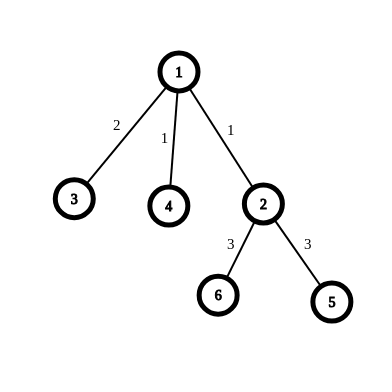点分治学习笔记

什么是点分治

$n>1000$ ，哦豁完蛋。

$n>10^4$ 后这种复杂度为 $O(n^2logn)$ 的做法也稳定TLE了，所以呢？

点分治原理

1. 在子树中，不经过根节点
2. 从一棵子树跨过根节点到另外一棵子树

选择重心

• $siz[i]$ 以 $i$ 为根节点的子树的节点数
• $sum$ 总结点数
• $f[i]$ 以 $i$ 为根节点的所有子树中最大子树的 $siz$

点分治的实现

• $vis[i]$ 用与记录节点 $i$ 是否被分治过

关于统计答案发生错误的那档事一些例题

4.[FJOI2014]最短路径树问题

思路

SB拼板题，套一个Dijkstra板子和一个点分治的板子就完了

0%